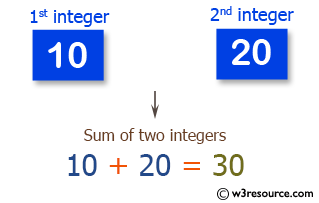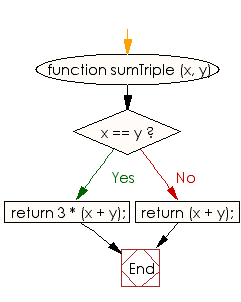# JavaScript: Compute the sum of the two given integers

## JavaScript Basic: Exercise-16 with Solution

Write a JavaScript program to compute the sum of the two given integers. If the two values are same, then returns triple their sum.

Pictorial Presentation:Sample Solution:

HTML Code:

``````<!DOCTYPE html>
<html>
<meta charset="utf-8">
<meta name="viewport" content="width=device-width">
<title>JavaScript program to compute the sum of the two given integers. If the two values are same, then return triple their sum</title>
<body>
</body>
</html>
```
```

JavaScript Code:

``````function sumTriple (x, y) {
if (x == y) {
return 3 * (x + y);
}
else
{
return (x + y);
}
}
console.log(sumTriple(10, 20));
console.log(sumTriple(10, 10));
```
```

Sample Output:

```30
60
```

Flowchart:ES6 Version:

``````function sumTriple (x, y) {
if (x == y) {
return 3 * (x + y);
}
else
{
return (x + y);
}
}
console.log(sumTriple(10, 20));
console.log(sumTriple(10, 10));
``````

Live Demo:

See the Pen JavaScript: Sum of two integers- basic-ex-16 by w3resource (@w3resource) on CodePen.

Improve this sample solution and post your code through Disqus

What is the difficulty level of this exercise?

Test your Programming skills with w3resource's quiz.

﻿

## JavaScript: Tips of the Day

Converts a string to title case

Example:

```const toTitleCase = str =>
str
.match(/[A-Z]{2,}(?=[A-Z][a-z]+[0-9]*|\b)|[A-Z]?[a-z]+[0-9]*|[A-Z]|[0-9]+/g)
.map(x => x.charAt(0).toUpperCase() + x.slice(1))
.join(' ');
console.log(toTitleCase('some_database_field_name')); // 'Some Database Field Name'
console.log(toTitleCase('Some label that needs to be title-cased')); // 'Some Label That Needs To Be Title Cased'
console.log(toTitleCase('some-package-name')); // 'Some Package Name'
console.log(toTitleCase('some-mixed_string with spaces_underscores-and-hyphens')); // 'Some Mixed String With Spaces Underscores And Hyphens'
```

Output:

```"Some Database Field Name"
"Some Label That Needs To Be Title Cased"
"Some Package Name"
"Some Mixed String With Spaces Underscores And Hyphens"
```